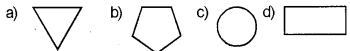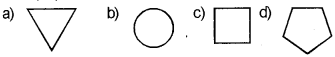# Kerala SSLC IT Theory Questions and Answers Chapter 4 Python Graphics

## Kerala Syllabus SSLC IT Theory Questions and Answers Chapter 4 Python Graphics

### Python Graphics Questions & Answers

Question 1.
From the following select one that is not a computer programming language
a) Python
b) C++
c) Java
d) Scanner
d) ScannerQuestion 2.
From the following select one computer programming language
a) Writer
b) GeoGebra
c) GIMP
d) Python
d) Python

Question 3.
What is the full form of IDE
a) Integrated Development Environment

Question 4.
From the following choose the IDE that are used to write programms in python language
a) IDLE
b) Geany
c) Turbo C++
d) VB IDE
a and b

Question 5.
Noramlly when you execute a general purpose program in IDLE, the output will be shown in window.
a) python shell window
b) python graphic window
c) python editor
d) None of these
a) python shell window

Question 6.
If the output a program written in python is graphical then it appears in a window
a) python shell window
b) python graphic window
c) python editor
d) None of these
b) python graphic window

Question 7.
From the following which in the associated software to create geometric shapes in python
a) Turtle
b) Writer
c) Calc
d) None of these
a) Turtle

Question 8.
Which command is used to add in the starting of a python program to work graphic commands
a) from turtle import
b) from input import
c) from Gimp import
d) None of these
a) from turtle import

Question 9.
From the following which command is equivalent to forward(100)
a) fw (100)
b) for (100)
c) fd (100)
d) fwd (100)
c) fd (100)

Question 10.
From the following which command is equivalent to right (90)
a) rt (90)
b) rgt (100)
c) rht (90)
d) rgt (90)
a) rt (90)

Question 11.
A loop statement contains another loop statement then it is called ………….
a) Nested loop
b) Direct loop
c) Indirect loop
d) None of these
a) Nested loop

Question 12.
What is the o/p of the following code snippet from turtle import for in range (3):
fd (100)
rt (120)a

Question 13.
What is the o/p of the following code snippet from turtle import*
for i in range (5):
fd (100)
rt (72)b

Question 14.
What is the o/p of the following code snippet from turtle import*
fori in range (4):
fd (100)
rt (90)c

Question 15.
………. command is used to give different colors to geometric shapes created in python graphic window
a) color ()
b) forward ()
c) right ()
d) left ()
a) color ()Question 16.
Which among the following is a loop statement?
a) for
b) print
c) home ()
d) iterate
a) for

Question 17.
What is the instructions used for removing everything from the Turtle Graphics window?
a) write ()
b) clear ()
c) home ()
d) iterate ()
b) clear ()

Question 18.
What is the purpose of the following instruction? Color (“black”, “red”)
a) For drawing geometric shape in black color and. filling it with red color
b) For drawing geometric shape in red color and filling it with black color
c) To fill a geometric shape with black and red color
d) The instruction is invalid
a) For drawing geometric shape in black color and filling it with red color

Question 19.
Match the following
1 – b
2 – c
3 – a

Question 20.
Choose the correct code segment from the following
a) for i in range ():
fd (100)
rt (90)
b) for i in range (4):
fd (100)
rt (90)
c) fori in range (4):
fd(100)
rt (90)
d) for i in range (4):
fd (100)
rt (90)
b) fori in range (4):
fd (100)
rt (90)

Question 21.
From the following choose the right command used to start filling colors to geometric shapes
a) begin_fill ()
b) start_fill ()
c) end_fill()
d) None of these
a) begin_fill ()

Question 22.
From the following choose the right command used to stop filling colours to geometric shapes
a) begin_fill ()
b) stop_fill ()
c) end_fill ()
d) Noneofthese
c) end_fill ()

Question 23.
Choose the correct code from the following
a) The instruction begin_fill () and end_fill () should be written in the same tab position
b) The instruction begin_fill () and end_fill () should not be written in the same tab position
c) Both a and b are correct
a) The instruction begin_fill () and end_fill () should be written in the same tab position

Question 24.
From the following which code is used for assigning four colours in variable clr
a) clr = [“blue”, “red”, “green”; “orange”]
b) clr = {blue, red, green, orange}
c) clr = (blue, red, green, orange)
d) Noneofthese
a) clr = [“blue”, “red”, “green”, “orange”]Question 25.
What is the use of the command fd ()
a) Move the Turtle to the right
b) Move the Turtle in a circle
c) Mark a point on the graphic screen
d) Specify the color of the line to be drawn on the screen
a) Move the Turtle to the right

Question 26.
What is the use of command circle ()?
a) Move the Turtle to the right
b) Move the Turtle in a circle
c) Mark a point on the graphic screen
d) Specify the color of the line to be drawn on the screen
b) Move the Turtle in a circle

Question 27.
What is the use of command dot ()?
a) Move the Turtle to the.right
b) Move the Turtle in a circle
c) Mark a point on the graphic screen
d) Specify the color of the line to be drawn on the screen
c) Mark a point on the graphic screen

Question 28.
What is the use of the command pen color ()?
a) Move the Turtle to the right
b) Move the Turtle in a circle
c) Mark a point on the graphic screen
d) Specify the color of the line to be drawn on the screen
d) Specify the color of the line to be drawn on the screen

Question 29.
What is the use of command pen size ()
a) Specify the thickness of the line to be drawn on the screen
b) To turn the turtle to the right according to given angle
c) To turn the turtle to the left according to the given angle
d) None of these
a) Specify the thickness of the line to be drawn on the screen

Question 30.
What is the use of command right ()?
a) Specify the thickness of the line to be drawn on the screen
b) To turn the turtle to the right according to given angle
c) To turn the turtle to the left according to the given angle
d) None of these